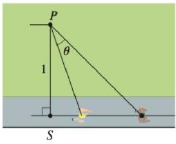Chapter 4.7, Problem 75E### Single Variable Calculus: Early Tr...

8th Edition
James Stewart
ISBN: 9781305270343

#### Solutions

Chapter
Section### Single Variable Calculus: Early Tr...

8th Edition
James Stewart
ISBN: 9781305270343
Textbook Problem

# An observer stands at a point P, one unit away from a track. Two runners start at the point S in the figure and ran along the track. One runner runs three times as fast as the other. Find the maximum value of the observer’s angle of sight θ between the runners.To determine

To find: The maximum value of the observer’s angle of sight θ between the runners.

Explanation

Given:

Distance between the point P from the track =1

Calculation:

Two runners start at the point S and run along the track.

One runner runs three times as fast as the other.

Let the distance of BC=3t.

Consider the triangle PSD,

Let, P=ψ

Consider the triangle PCD

P=θ

Consider the triangle PSC

P=θ+ψ

Then,

tan(θ+ψ)=3t1tanθ+tanψ1tanθtanψ=3tt+tanθ1ttanθ=3t

Simplify further,

3t(1ttanθ)=t+tanθ2t=(1+3t2)tanθtanθ=2t1+3t2

Let f(t)=tanθ=2t1+3t2

Differentiate with respect to t,

dfdt=2(1+3t2)2t(6t)(1+3t2)2=2(13t2)(1+3t2)2

For critical points, dfdt=0

2(13t2)(1+3t2)2=013t2=0t=±13

Differentiate dfdt with respect to t,

d2fdt2=(1+3t2)2(12t)2(1

### Still sussing out bartleby?

Check out a sample textbook solution.

See a sample solution

#### The Solution to Your Study Problems

Bartleby provides explanations to thousands of textbook problems written by our experts, many with advanced degrees!

Get Started

#### Find more solutions based on key concepts